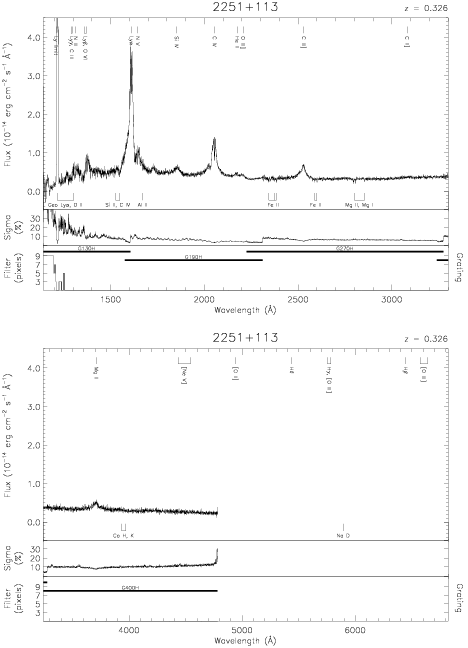Download dataset
TELESCOP= 'HST     '
INSTRUME= 'FOS     '
EQUINOX =               2000.0
DATE-OBS= '1991-09-06T14:16:54'
RA_PNT  =       343.5433516343
DEC_PNT =        11.6108302832
PA_PNT  =       203.8131408693
OBJECT  = '2251+113'
ROOTNM01= 'Y0PE0P02T'
GRNDMD01= 'SPECTROSCOPY'
DETECT01= 'BLUE    '
APERID01= 'A-1     '
FGWAID01= 'H13     '
ROOTNM02= 'Y0PE0P03T'
GRNDMD02= 'SPECTROSCOPY'
DETECT02= 'BLUE    '
APERID02= 'A-1     '
FGWAID02= 'H19     '
ROOTNM03= 'Y0PE0Q02T'
GRNDMD03= 'SPECTROSCOPY'
DETECT03= 'AMBER   '
APERID03= 'A-1     '
FGWAID03= 'H27     '
ROOTNM04= 'Y0PE0Q03T'
GRNDMD04= 'SPECTROSCOPY'
DETECT04= 'AMBER   '
APERID04= 'A-1     '
FGWAID04= 'H40     '
ROOTNM05= 'Y0RV0H03T'
DETECT05= 'AMBER   '
APERID05= 'C-2     '
FGWAID05= 'H19     '
ROOTNM06= 'Y0RV0H04T'
DETECT06= 'AMBER   '
APERID06= 'C-2     '
FGWAID06= 'H19     '
ROOTNM07= 'Y0RV0H05T'
DETECT07= 'AMBER   '
APERID07= 'C-2     '
FGWAID07= 'H19     '
ROOTNM08= 'Y0RV0H06T'
DETECT08= 'AMBER   '
APERID08= 'C-2     '
FGWAID08= 'H27     '
ROOTNM09= 'Y1450K03T'
DETECT09= 'BLUE    '
APERID09= 'C-2     '
FGWAID09= 'H13     '
ROOTNM10= 'Y1450K04T'
DETECT10= 'BLUE    '
APERID10= 'C-2     '
FGWAID10= 'H13     '
ROOTNM11= 'Y1450K05T'
DETECT11= 'BLUE    '
APERID11= 'C-2     '
FGWAID11= 'H13     '
ROOTNM12= 'Y1450K06T'
DETECT12= 'BLUE    '
APERID12= 'C-2     '
FGWAID12= 'H13     '
ROOTNM13= 'Y1450K07T'